# 8.5 Conic sections in polar coordinates  (Page 6/8)

 Page 6 / 8

$\frac{{x}^{2}}{9}-\frac{{y}^{2}}{16}=1$

$\frac{{\left(y-1\right)}^{2}}{49}-\frac{{\left(x+1\right)}^{2}}{4}=1$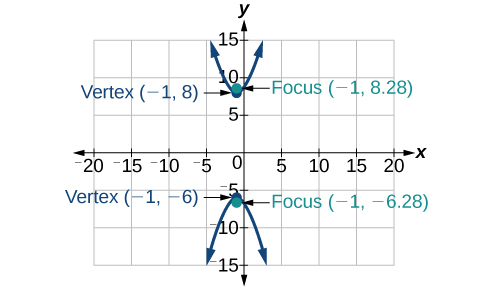${x}^{2}-4{y}^{2}+6x+32y-91=0$

$2{y}^{2}-{x}^{2}-12y-6=0$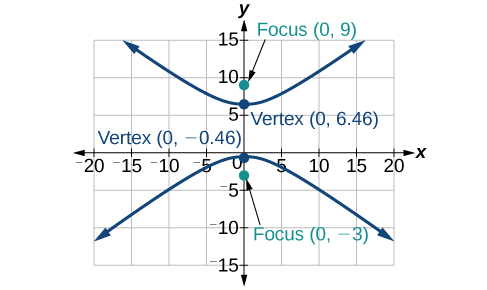For the following exercises, find the equation of the hyperbola.

Center at $\text{\hspace{0.17em}}\left(0,0\right),$ vertex at $\text{\hspace{0.17em}}\left(0,4\right),$ focus at $\text{\hspace{0.17em}}\left(0,-6\right)$

Foci at $\text{\hspace{0.17em}}\left(3,7\right)\text{\hspace{0.17em}}$ and $\text{\hspace{0.17em}}\left(7,7\right),$ vertex at $\text{\hspace{0.17em}}\left(6,7\right)$

$\frac{{\left(x-5\right)}^{2}}{1}-\frac{{\left(y-7\right)}^{2}}{3}=1$

## The Parabola

For the following exercises, write the equation of the parabola in standard form. Then give the vertex, focus, and directrix.

${y}^{2}=12x$

${\left(x+2\right)}^{2}=\frac{1}{2}\left(y-1\right)$

${\left(x+2\right)}^{2}=\frac{1}{2}\left(y-1\right);\text{\hspace{0.17em}}$ vertex: $\text{\hspace{0.17em}}\left(-2,1\right);\text{\hspace{0.17em}}$ focus: $\text{\hspace{0.17em}}\left(-2,\frac{9}{8}\right);\text{\hspace{0.17em}}$ directrix: $\text{\hspace{0.17em}}y=\frac{7}{8}$

${y}^{2}-6y-6x-3=0$

${x}^{2}+10x-y+23=0$

${\left(x+5\right)}^{2}=\left(y+2\right);\text{\hspace{0.17em}}$ vertex: $\text{\hspace{0.17em}}\left(-5,-2\right);\text{\hspace{0.17em}}$ focus: $\text{\hspace{0.17em}}\left(-5,-\frac{7}{4}\right);\text{\hspace{0.17em}}$ directrix: $\text{\hspace{0.17em}}y=-\frac{9}{4}$

For the following exercises, graph the parabola, labeling vertex, focus, and directrix.

${x}^{2}+4y=0$

${\left(y-1\right)}^{2}=\frac{1}{2}\left(x+3\right)$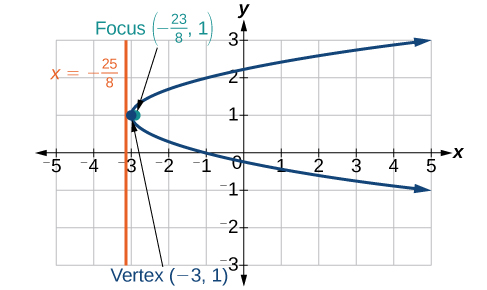${x}^{2}-8x-10y+46=0$

$2{y}^{2}+12y+6x+15=0$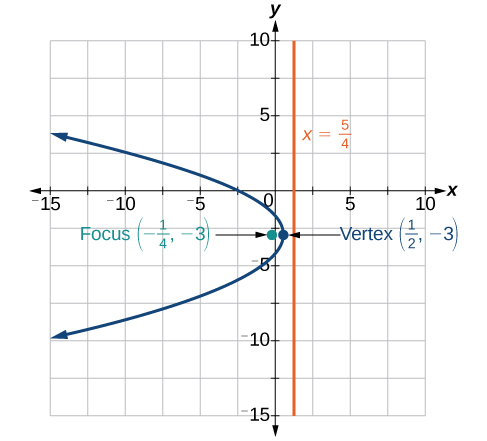For the following exercises, write the equation of the parabola using the given information.

Focus at $\text{\hspace{0.17em}}\left(-4,0\right);\text{\hspace{0.17em}}$ directrix is $\text{\hspace{0.17em}}x=4$

Focus at $\text{\hspace{0.17em}}\left(2,\frac{9}{8}\right);\text{\hspace{0.17em}}$ directrix is $\text{\hspace{0.17em}}y=\frac{7}{8}$

${\left(x-2\right)}^{2}=\left(\frac{1}{2}\right)\left(y-1\right)$

A cable TV receiving dish is the shape of a paraboloid of revolution. Find the location of the receiver, which is placed at the focus, if the dish is 5 feet across at its opening and 1.5 feet deep.

## Rotation of Axes

For the following exercises, determine which of the conic sections is represented.

$16{x}^{2}+24xy+9{y}^{2}+24x-60y-60=0$

${B}^{2}-4AC=0,$ parabola

$4{x}^{2}+14xy+5{y}^{2}+18x-6y+30=0$

$4{x}^{2}+xy+2{y}^{2}+8x-26y+9=0$

${B}^{2}-4AC=-31<0,$ ellipse

For the following exercises, determine the angle $\text{\hspace{0.17em}}\theta \text{\hspace{0.17em}}$ that will eliminate the $\text{\hspace{0.17em}}xy\text{\hspace{0.17em}}$ term, and write the corresponding equation without the $\text{\hspace{0.17em}}xy\text{\hspace{0.17em}}$ term.

${x}^{2}+4xy-2{y}^{2}-6=0$

${x}^{2}-xy+{y}^{2}-6=0$

$\theta ={45}^{\circ },{{x}^{\prime }}^{2}+3{{y}^{\prime }}^{2}-12=0$

For the following exercises, graph the equation relative to the $\text{\hspace{0.17em}}{x}^{\prime }{y}^{\prime }\text{\hspace{0.17em}}$ system in which the equation has no $\text{\hspace{0.17em}}{x}^{\prime }{y}^{\prime }\text{\hspace{0.17em}}$ term.

$9{x}^{2}-24xy+16{y}^{2}-80x-60y+100=0$

${x}^{2}-xy+{y}^{2}-2=0$

$\theta ={45}^{\circ }$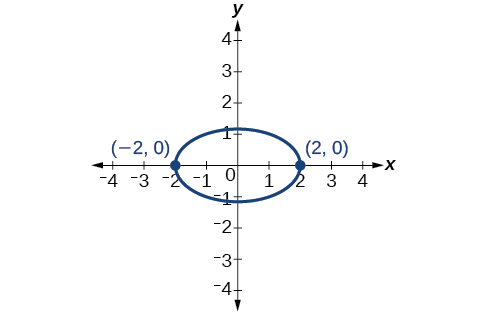$6{x}^{2}+24xy-{y}^{2}-12x+26y+11=0$

## Conic Sections in Polar Coordinates

For the following exercises, given the polar equation of the conic with focus at the origin, identify the eccentricity and directrix.

Hyperbola with $\text{\hspace{0.17em}}e=5\text{\hspace{0.17em}}$ and directrix $\text{\hspace{0.17em}}2\text{\hspace{0.17em}}$ units to the left of the pole.

Ellipse with $\text{\hspace{0.17em}}e=\frac{3}{4}\text{\hspace{0.17em}}$ and directrix $\text{\hspace{0.17em}}\frac{1}{3}\text{\hspace{0.17em}}$ unit above the pole.

For the following exercises, graph the conic given in polar form. If it is a parabola, label the vertex, focus, and directrix. If it is an ellipse or a hyperbola, label the vertices and foci.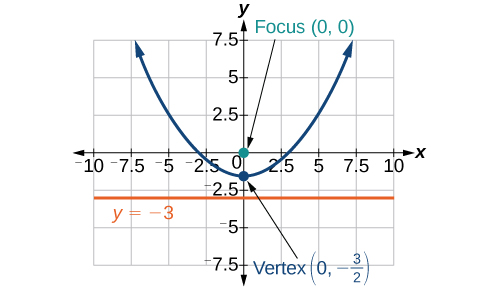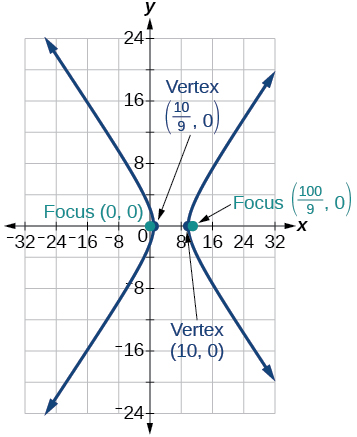For the following exercises, given information about the graph of a conic with focus at the origin, find the equation in polar form.

Directrix is $\text{\hspace{0.17em}}x=3\text{\hspace{0.17em}}$ and eccentricity $\text{\hspace{0.17em}}e=1$

Directrix is $\text{\hspace{0.17em}}y=-2\text{\hspace{0.17em}}$ and eccentricity $\text{\hspace{0.17em}}e=4$

## Practice test

For the following exercises, write the equation in standard form and state the center, vertices, and foci.

$\frac{{x}^{2}}{9}+\frac{{y}^{2}}{4}=1$

$\frac{{x}^{2}}{{3}^{2}}+\frac{{y}^{2}}{{2}^{2}}=1;\text{\hspace{0.17em}}$ center: $\text{\hspace{0.17em}}\left(0,0\right);\text{\hspace{0.17em}}$ vertices: $\text{\hspace{0.17em}}\left(3,0\right),\left(–3,0\right),\left(0,2\right),\left(0,-2\right);\text{\hspace{0.17em}}$ foci: $\left(\sqrt{5},0\right),\left(-\sqrt{5},0\right)$

$9{y}^{2}+16{x}^{2}-36y+32x-92=0$

For the following exercises, sketch the graph, identifying the center, vertices, and foci.

$\frac{{\left(x-3\right)}^{2}}{64}+\frac{{\left(y-2\right)}^{2}}{36}=1$

center: $\text{\hspace{0.17em}}\left(3,2\right);\text{\hspace{0.17em}}$ vertices: $\text{\hspace{0.17em}}\left(11,2\right),\left(-5,2\right),\left(3,8\right),\left(3,-4\right);\text{\hspace{0.17em}}$ foci: $\text{\hspace{0.17em}}\left(3+2\sqrt{7},2\right),\left(3-2\sqrt{7},2\right)$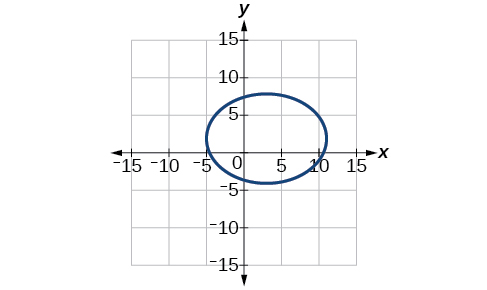$2{x}^{2}+{y}^{2}+8x-6y-7=0$

Write the standard form equation of an ellipse with a center at $\text{\hspace{0.17em}}\left(1,2\right),$ vertex at $\text{\hspace{0.17em}}\left(7,2\right),$ and focus at $\text{\hspace{0.17em}}\left(4,2\right).$

$\frac{{\left(x-1\right)}^{2}}{36}+\frac{{\left(y-2\right)}^{2}}{27}=1$

A whispering gallery is to be constructed with a length of 150 feet. If the foci are to be located 20 feet away from the wall, how high should the ceiling be?

For the following exercises, write the equation of the hyperbola in standard form, and give the center, vertices, foci, and asymptotes.

$\frac{{x}^{2}}{49}-\frac{{y}^{2}}{81}=1$

$\frac{{x}^{2}}{{7}^{2}}-\frac{{y}^{2}}{{9}^{2}}=1;\text{\hspace{0.17em}}$ center: $\text{\hspace{0.17em}}\left(0,0\right);\text{\hspace{0.17em}}$ vertices $\text{\hspace{0.17em}}\left(7,0\right),\left(-7,0\right);\text{\hspace{0.17em}}$ foci: $\text{\hspace{0.17em}}\left(\sqrt{130},0\right),\left(-\sqrt{130},0\right);\text{\hspace{0.17em}}$ asymptotes: $\text{\hspace{0.17em}}y=±\frac{9}{7}x$

$16{y}^{2}-9{x}^{2}+128y+112=0$

For the following exercises, graph the hyperbola, noting its center, vertices, and foci. State the equations of the asymptotes.

$\frac{{\left(x-3\right)}^{2}}{25}-\frac{{\left(y+3\right)}^{2}}{1}=1$

center: $\text{\hspace{0.17em}}\left(3,-3\right);\text{\hspace{0.17em}}$ vertices: $\text{\hspace{0.17em}}\left(8,-3\right),\left(-2,-3\right);$ foci: $\text{\hspace{0.17em}}\left(3+\sqrt{26},-3\right),\left(3-\sqrt{26},-3\right);\text{\hspace{0.17em}}$ asymptotes: $\text{\hspace{0.17em}}y=±\frac{1}{5}\left(x-3\right)-3$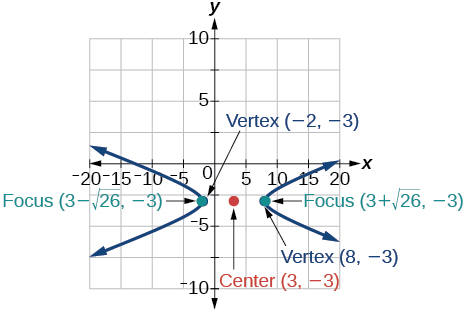${y}^{2}-{x}^{2}+4y-4x-18=0$

Write the standard form equation of a hyperbola with foci at $\text{\hspace{0.17em}}\left(1,0\right)\text{\hspace{0.17em}}$ and $\text{\hspace{0.17em}}\left(1,6\right),$ and a vertex at $\text{\hspace{0.17em}}\left(1,2\right).$

$\frac{{\left(y-3\right)}^{2}}{1}-\frac{{\left(x-1\right)}^{2}}{8}=1$

For the following exercises, write the equation of the parabola in standard form, and give the vertex, focus, and equation of the directrix.

${y}^{2}+10x=0$

$3{x}^{2}-12x-y+11=0$

${\left(x-2\right)}^{2}=\frac{1}{3}\left(y+1\right);\text{\hspace{0.17em}}$ vertex: $\text{\hspace{0.17em}}\left(2,-1\right);\text{\hspace{0.17em}}$ focus: $\text{\hspace{0.17em}}\left(2,-\frac{11}{12}\right);\text{\hspace{0.17em}}$ directrix: $\text{\hspace{0.17em}}y=-\frac{13}{12}$

For the following exercises, graph the parabola, labeling the vertex, focus, and directrix.

${\left(x-1\right)}^{2}=-4\left(y+3\right)$

${y}^{2}+8x-8y+40=0$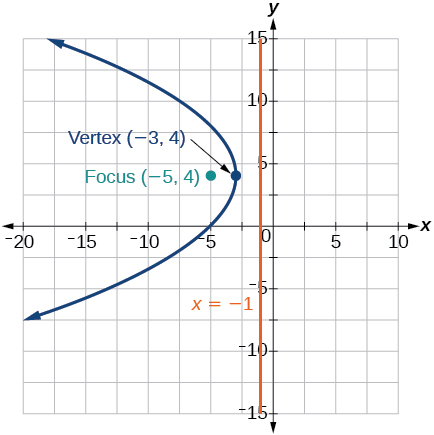Write the equation of a parabola with a focus at $\text{\hspace{0.17em}}\left(2,3\right)\text{\hspace{0.17em}}$ and directrix $\text{\hspace{0.17em}}y=-1.$

A searchlight is shaped like a paraboloid of revolution. If the light source is located 1.5 feet from the base along the axis of symmetry, and the depth of the searchlight is 3 feet, what should the width of the opening be?

Approximately $\text{\hspace{0.17em}}8.49\text{\hspace{0.17em}}$ feet

For the following exercises, determine which conic section is represented by the given equation, and then determine the angle $\text{\hspace{0.17em}}\theta \text{\hspace{0.17em}}$ that will eliminate the $\text{\hspace{0.17em}}xy\text{\hspace{0.17em}}$ term.

$3{x}^{2}-2xy+3{y}^{2}=4$

${x}^{2}+4xy+4{y}^{2}+6x-8y=0$

parabola; $\text{\hspace{0.17em}}\theta \approx {63.4}^{\circ }$

For the following exercises, rewrite in the $\text{\hspace{0.17em}}{x}^{\prime }{y}^{\prime }\text{\hspace{0.17em}}$ system without the $\text{\hspace{0.17em}}{x}^{\prime }{y}^{\prime }\text{\hspace{0.17em}}$ term, and graph the rotated graph.

$11{x}^{2}+10\sqrt{3}xy+{y}^{2}=4$

$16{x}^{2}+24xy+9{y}^{2}-125x=0$

${{x}^{\prime }}^{2}-4{x}^{\prime }+3{y}^{\prime }=0$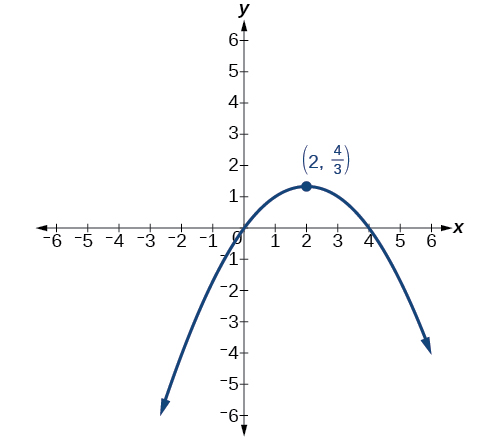For the following exercises, identify the conic with focus at the origin, and then give the directrix and eccentricity.

Hyperbola with $\text{\hspace{0.17em}}e=\frac{3}{2},\text{\hspace{0.17em}}$ and directrix $\text{\hspace{0.17em}}\frac{5}{6}\text{\hspace{0.17em}}$ units to the right of the pole.

For the following exercises, graph the given conic section. If it is a parabola, label vertex, focus, and directrix. If it is an ellipse or a hyperbola, label vertices and foci.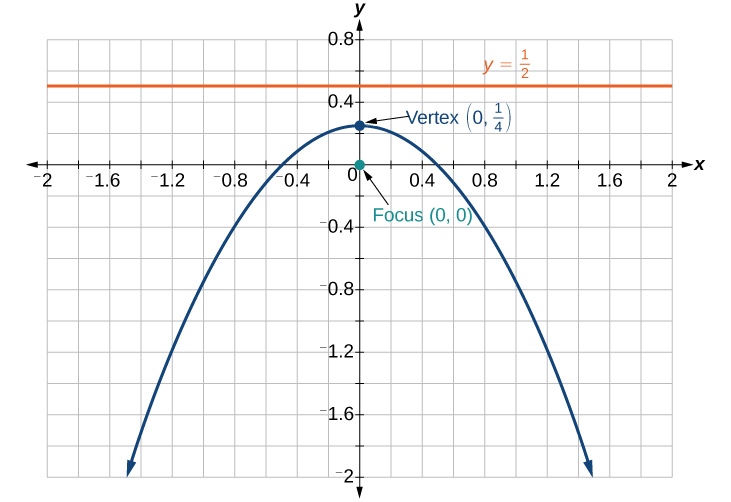Find a polar equation of the conic with focus at the origin, eccentricity of $\text{\hspace{0.17em}}e=2,$ and directrix: $\text{\hspace{0.17em}}x=3.$

how do I set up the problem?
what is a solution set?
Harshika
find the subring of gaussian integers?
Rofiqul
hello, I am happy to help!
Abdullahi
hi mam
Mark
find the value of 2x=32
divide by 2 on each side of the equal sign to solve for x
corri
X=16
Michael
Want to review on complex number 1.What are complex number 2.How to solve complex number problems.
Beyan
yes i wantt to review
Mark
use the y -intercept and slope to sketch the graph of the equation y=6x
how do we prove the quadratic formular
Darius
hello, if you have a question about Algebra 2. I may be able to help. I am an Algebra 2 Teacher
thank you help me with how to prove the quadratic equation
Seidu
may God blessed u for that. Please I want u to help me in sets.
Opoku
what is math number
4
Trista
x-2y+3z=-3 2x-y+z=7 -x+3y-z=6
can you teacch how to solve that🙏
Mark
Solve for the first variable in one of the equations, then substitute the result into the other equation. Point For: (6111,4111,−411)(6111,4111,-411) Equation Form: x=6111,y=4111,z=−411x=6111,y=4111,z=-411
Brenna
(61/11,41/11,−4/11)
Brenna
x=61/11 y=41/11 z=−4/11 x=61/11 y=41/11 z=-4/11
Brenna
Need help solving this problem (2/7)^-2
x+2y-z=7
Sidiki
what is the coefficient of -4×
-1
Shedrak
the operation * is x * y =x + y/ 1+(x × y) show if the operation is commutative if x × y is not equal to -1
An investment account was opened with an initial deposit of \$9,600 and earns 7.4% interest, compounded continuously. How much will the account be worth after 15 years?
lim x to infinity e^1-e^-1/log(1+x)
given eccentricity and a point find the equiationByByByBy Abby SharpBy OpenStaxBy OpenStaxBy OpenStaxBy OpenStaxBy Yasser IbrahimBy OpenStaxBy OpenStaxBy